Depth of Field

# Depth of Field### Basics

When a lens focuses on a subject at a distance, all subjects at that distance are sharply focused. Subjects that are not at the same distance are out-of-focus and theoretically are not sharp. However, since human eyes cannot distinguish very small degree of unsharpness, some subjects that are in front of and behind the in-focus subjects can still appear sharp. The zone of acceptable sharpness is referred to as the depth of field. Thus, increasing the depth of field increases the sharpness of an image. We can use smaller aperture to increase the depth of field.

The following shows an example. The lens focuses at the middle between the 3 inch and 4 inch marks. Thus, the 3 inch and 4 inch marks are sharp in all images. The 5 inch mark is not very sharp at F3.2, and its sharpness is improved as the lens closes down to F3.6. Then, it becomes sharp in all subsequent images. The 6 inch and 7 inch marks are not sharp until F5.0 and F6.4, respectively. The 8 inch mark becomes reasonably sharp when the lens closes down to F8.0. The 9 inch and 10 inch marks are not sharp in all images; but, they become sharper as the lens closes down. For the foreground, the 2 inch mark is acceptable at F3.2 and becomes in-focus at F4.0. The 1 inch mark is not sharp until F5.6, and the lead of the ruler becomes reasonably sharp at F7.1. As you can see, the range of sharpness (i.e., depth of field) gets larger as the aperture gets smaller. Therefore, use a smaller aperture if a greater depth of field is needed. Please check the Aperture-Priority Mode to learn more about the use of aperture and its impact on depth of field.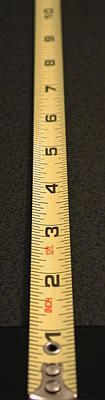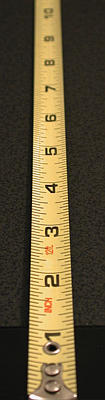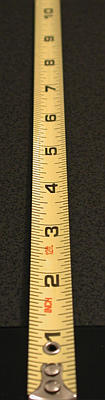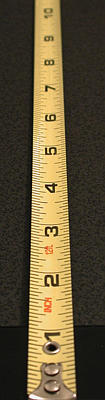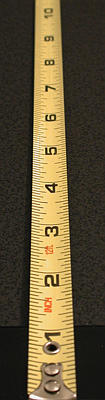F3.2 F3.6 F4.0 F4.5 F5.0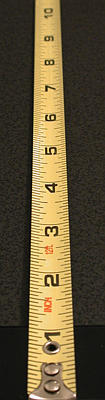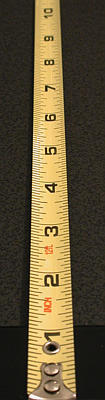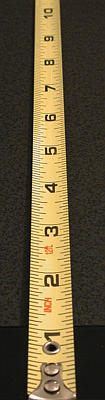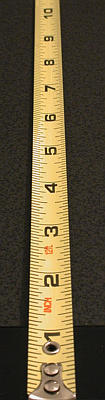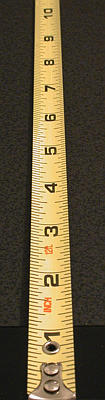F5.6 F6.4 F7.1 F8.0 F9.0 Click on the image to see a larger one

### Why?

Suppose the lens focuses on the yellow dot as shown in the figure below. This subject generates a yellow dot on the image plane. Once focused, all subjects that have the same subject-lens distance as that of the yellow dot will appear sharp. Now, consider a white dot that is behind the yellow dot (i.e., with larger subject-lens distance). Since it is out-of-focus, it will not produce a sharp white dot image. Instead, its image is formed somewhere in front of the image plane. On the image plane, the image of this white dot is a circle as shown below. This circle is usually referred to as a circle of confusion. As the subject-lens distance increases, the size of this circle increases. The same holds true for a subject in front of the yellow dot (e.g., the green dot in the figure below). Since these circles of confusion are actually out-of-focus images of dots, if we can reduce the size of circle of confusion, we can increase the sharpness of the resulting image. But, how?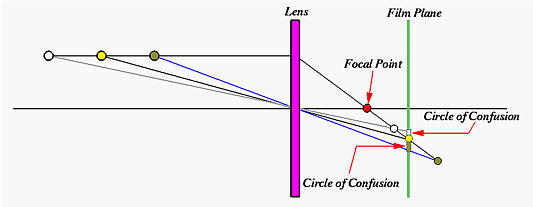It turns out to be very simple. Since circles of confusion are formed by light rays passing through the lens tube, the size of a circle of confusion is proportional to the amount of light that can pass through the lens tube. This means smaller (resp., larger) circles of confusion will be formed if less (resp., more) light can pass through. Restricting how much light can pass through the lens is the function of aperture in the lens tube. Therefore, a smaller aperture means allowing less light to reach the image capturing device, which means we have smaller circles of confusion, and, as a result, we have a sharper image!

### Hyperfocal Distance

In addition to closing down the aperture, we can also focus at the hyperfocal distance to maximize depth of field. If a lens focuses at infinity, the depth of field starts at somewhere in front of the lens and extends to infinity. More precisely, from that point on, the scene appears sharp, and subjects between that point and the lens are out-of-focus. The distance from the lens to that point is referred to as the hyperfocal distance. In the figure below, the hyperfocal distance is marked with a thick red line.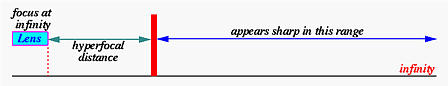After determining the hyperfocal distance, we can point the camera at this distance. The resulting depth of field starts from halfway of the hyperfocal distance and extends to infinity. This is, in fact, the maximum depth of field we can get. The concept is shown in the figure below.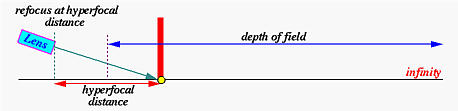Why is this hyperfocal distance important? When shooting a distant subject or scene, many people point the camera to that subject(s). While the result may be satisfactory, in many cases the foreground looks out-of-focus. This is, of course, because of insufficient depth of field. Should this happen, the above technique becomes very useful: (1) focus at infinity; (2) determine the hyperfocal distance; (3) refocus at the hyperfocal distance; and (4) take a photo.

### A Reminder

Hyperfocal distance is a function of the aperture being used. Changing aperture changes hyperfocal distance.

### Can We Use the Smallest Aperture to Yield Greater Depth of Field?

Unfortunately, in general, the answer is "no." As the light rays passing the lens tube and the aperture blades, some may be diffracted as shown in the figures below. If the aperture is large, because the proportion of the diffracted light and the non-diffracted light is so small and is negligible, diffraction does not contribute to the loss-of-quality very much. See the left figure below. However, when aperture is small, the amount of light that can pass through the aperture is reduced and hence the proportion of the diffracted and non-diffracted light becomes significant. As a result, the quality of the image is also reduced. See the right figure below. Therefore, closing the aperture all the way down to the smallest may not always increase the quality of an image. In general, the quality of a lens increases as the aperture closes down. This improvement will reach certain point. After this, quality goes down because of the impact of diffraction.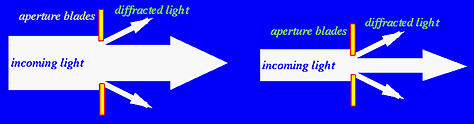### An Important Note

Discussion in this section is based on an ideal lens and is somewhat simplified and theoretical. Since camera lenses are not perfect and may be different from manufacturer to manufacturer and even from lens to lens of the same type made by the same manufacture, depth of field calculation is not so simple.

#### Circles of Confusion: Revisited

Recall from the concept of circle of confusion that the resolving power of human eyes is not very high, and, as a result, some sizes of circle of confusion may be perceived as perfect points. Thus, to understand the calculation of depth of field, we need to know what is the maximum size of circle of confusion that would be treated as a point by human eyes. Unfortunately, this maximum size, or diameter, of circle of confusion depends on so many factors. The most important fact is certainly the image size.

Suppose the same scene is recorded using two lenses, each of which is used on a camera with a different image size. If both lenses produce the same size of circle of confusion and both images are enlarged to the same size, then it is obvious that the smaller image requires higher magnification to bring its size equal to that of the larger image. As a result, those circles of confusion recorded on the smaller image will become larger in the enlarged image than those from the larger image. Of course, the enlarged image from the smaller image will not look as sharp as the one from the larger image. This is why enlargements from large format camera always look sharper than the enlargements from smaller format cameras. Thus, this is the major reason that image size has to be a factor in the determination of the maximum size of circle of confusion.

When Leica started the 24 × 36 mm images on 35mm film, they found out that a suitable size of the circle of confusion on the film for the Leica 50mm lens should be about 1/30mm. Later, the international standards defines the maximum diameter of permissible circle of confusion, as showns below, to be an acceptable value based on the film technology of pre-World War II. This value is approximately equivalent to the resolution power of 30 lines per millimeter (i.e., 30 lp/mm). However, today's high resolution film could have a resolution power as high as or even higher than 200 lp/mm, and, hence, the following definition is out-of-date. Consequently, modern lenses will have their diameters smaller than the values computed with the following formula. For example, Carl Zeiss found out that using 1500 as the divisor would be tolerable for 35mm format. Since the diagonal length of 35mm film is 43.27mm, the diameter is 0.029mm. However, since lens manufacturers never publish the diameter of circle of confusion for their lenses, we have no way to obtain an exact value. What we do know is that lens manufacturers will use a smaller but not too small size in the design of their lenses.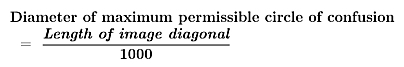The case for digital cameras is a little different. The 4500's CCD has a diagonal of 1/1.8" = 14.11mm. Although it is the diagonal of the complete CCD rather than the diagonal of the area for recording the captured image, the difference is small. Without loss of generality, we just use 14.11mm as the length of the diagonal. Hence, the maximum diameter of the circle of confusion is 0.009mm. Some prefer to use Leica's original definition that the diameter of the maximum permissible circle of confusion if the quotient of diving the focal length of the standard lens by 1500. Why standard lens? It is because when Leica created the 24×36 format, Leica's camera was fit with a 50mm Leica lens! On the 4500, the equivalent 50mm focal length is 10.3mm. Dividing 10.3mm by 1500 yields the diameter of 0.0069. Therefore, some that 0.006mm should be used. Given the diagonal of 14.11mm, the width and height in the 4:3 aspect ratio is 11.29mm and 8.47mm, respectively. The 4500 CCD can record an image of 2272 x 1704. Thus, the dimension of a pixel is no more than 11.29mm/2272 = 0.00497. Consequently, a circle of confusion of diameter 0.006mm covers a little more than one pixel, which is reasonable. The following table lists the diameters of a number of different formats for your reference, where 0.03mm and 0.006mm are used for 35mm film and 4500, respectively.

 Format Diameter 4500 0.006mm 35mm 0.03mm 6×6 cm 0.056mm 4×5 inch 0.11mm

#### Hyperfocal Distance: Revisited

The hyperfocal distance of a lens at a given focal length and aperture is calculated as follows. Note that the diameter of maximum permissible circle of confusion has to be chosen properly because the same lens used for a different image size gives a different diameter value.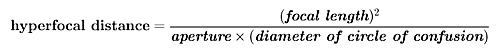From this formula, we learn the following:

1. A longer focal length produces a larger hyperfocal distance. This means that the closest focusing distance of a shorter focal length lens is shorter than that of a longer focal length lens, and covers a larger depth.".
2. The smaller the aperture, the shorter the hyperfocal distance. This means that a smaller aperture produces larger depth of field.
3. The larger the maximum permissible circle of confusion, the shorter the hyperfocal distance. This means that if human eyes can treat a larger circle of confusion as a point, the hyperfocal distance will be closer to the camera.
Let us compare the hyperfocal distance of 4500 and a 35mm lens. Suppose we fix the focal length of a 4500 at 8mm, which is equivalent to 38mm of a 35mm lens. Here is the computed results, in mm.

 Aperture F2.8 F5.6 F8.0 F11.0 4500 @ 8mm 3810 1905 1333 970 35mm lens @ 38mm 17190 8595 6017 4370

In fact, it is not difficult to verify that under this setting, the 35mm lens has a hyperfocal distance 4.5 times farther than that of 4500. This explains partially that why images obtained with 4500 always look sharper than those using 35mm lenses.

#### Depth of Field: Revisited

Depth of field is bounded by two distance measures: near and far, where near is the closest distance (to the camera) that subjects appear to be sharp and far is the shortest distance beyond which subjects look out-of-focus (or longest distance within which subjects appear to be in-focus). Thus, subjects with subject distances between near and far appear sharp on the resulting image. Of course, near must be less than far, and subject distances lies in between. The following shows the computation formulae.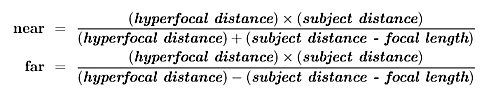Variables involved are focal length, subject distance (i.e., the distance between the subject and the camera), and hyperfocal distance. Note that aperture does not occur explicitly because it is used in the computation of hyperfocal distance. Therefore, we should not naively conclude that aperture is not used in the computation of depth of field.

There is one more important note. The subject distance does not have to be larger than the hyperfocal distance, because once we focus at the hyperfocal distance (i.e., subject distance = hyperfocal distance), we will have maximum depth of field, and near is half of the hyperfocal distance and far is at infinity.

Let us take a look at an example. Let the 4500 use focal length 8mm (38mm, 35mm equivalent) at aperture F5.6. Note that the hyperfocal distance is 1143mm from the above table. If the lens is focused at 100mm (10cm), we have near = 48mm and far = 109mm; if the lens is focused at 1000mm (1m), we have near = 535mm and far = 7570mm. On the other hand, if the lens is a 35mm lens of focal length 38mm (equivalent to 4500's 8mm) at the same aperture F5.6, the above table gives the hyperfocal distance 5157mm. If the lens is focused at 100mm (assuming it is possible), we have near = 99mm and far = 101mm; if the lens is focused at 1000mm, we have near = 842mm and far = 1229mm. The following table summarizes these information:

 Subject dist = 100mm Subject Dist = 1000mm Camera Near Far Length Near Far Length 4500 @ 8mm and F5.6 95 105 10 658 2087 1429 35mm lens @ 38mm and F5.6 99 101 2 899 1126 227
 All values have unit mm

From this table, it is easily seen that 4500's short focal length produces a much longer area within which subjects appear sharp than an equivalent 35mm lens can produce. This is why images obtained from a 4500 appear to be sharper. The crucial part is image size which makes the diameter of maximum permissible circle of confusion much smaller than the 35mm counterpart! This is also the reason that you cannot obtain a blurred background that is usually produced by a 35mm lens using larger apertures.